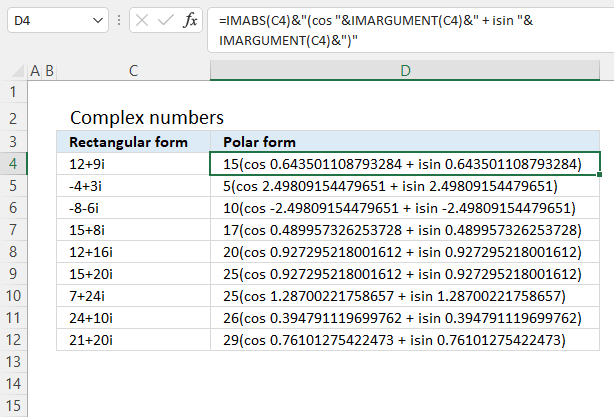Author: Oscar Cronquist Article last updated on October 27, 2022Formula in cell D4:

=IMABS(C4)&"(cos "&IMARGUMENT(C4)&" + isin "&IMARGUMENT(C4)&")"

Complex numbers are usually presented in this form

z = x + yi

or

z = x + yj

However, complex numbers can also be represented in polar form:

z = r*(cos θ + isin θ)

In other words, theta θ in the polar form is calculated using the IMARGUMENT based on complex numbers.

Pythagorean Theorem

r= x2 + y2

To calculate the absolute value we can use this formula:

r = √(x2+y2)

Excel has a function that does this for you, the IMABS function calculates the absolute value based on complex numbers.

### Explaining formula

#### Step 1 - Calculate theta θ

The IMARGUMENT function calculates theta θ which is an angle displayed in radians based on complex numbers in rectangular form.

Function syntax: IMARGUMENT(inumber)

IMARGUMENT(C4)

becomes

IMARGUMENT("12+9i")

and returns

0.643501108793284

#### Step 2 - Calculate the absolute value

The IMABS function calculates the absolute value (modulus) of a complex number in x + yi or x + yj text format.

Function syntax: IMABS(inumber)

IMABS(C4)

becomes

IMABS("12+9i")

and returns

15

#### Step 3 - Join calculations with text

The ampersand character lets you concatenate values in an Excel Formula.

IMABS(C4)&"(cos "&IMARGUMENT(C4)&" + isin "&IMARGUMENT(C4)&")"

#### Step 4 - Evaluate the formula

IMABS(C4)&"(cos "&IMARGUMENT(C4)&" + isin "&IMARGUMENT(C4)&")"

becomes

15&"(cos "&0.643501108793284&" + isin "&0.643501108793284&")"

and returns

15(cos 0.643501108793284 + isin 0.643501108793284)